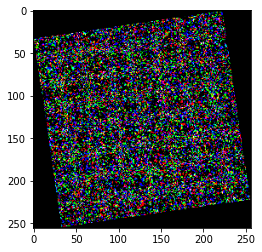# Adding further transformation to preset_transformations

I have loaded the resnet50’s transformations from the weights

``````resnet_50_weights = ResNet50_Weights.IMAGENET1K_V2
resnet_50_model = resnet50(weights=resnet_50_weights)
resnet_50_transformations = resnet_50_weights.transforms()
``````

However I would like to add another transformation to this, say `transforms.RandomRotation(15, expand=True)` How would I add this. Using Compose threw an error

It seems to work for me:

``````resnet_50_weights = models.resnet.ResNet50_Weights.IMAGENET1K_V2
resnet_50_transformations = resnet_50_weights.transforms()

trans = transforms.Compose([
resnet_50_transformations,
transforms.RandomRotation(15, expand=True)
])

x = torch.randn(3, 224, 224)

ref = resnet_50_transformations(x)
out = trans(x)

plt.imshow(ref.permute(1, 2, 0).numpy())
plt.imshow(out.permute(1, 2, 0).numpy())
``````

Output:
`ref`:`out`: